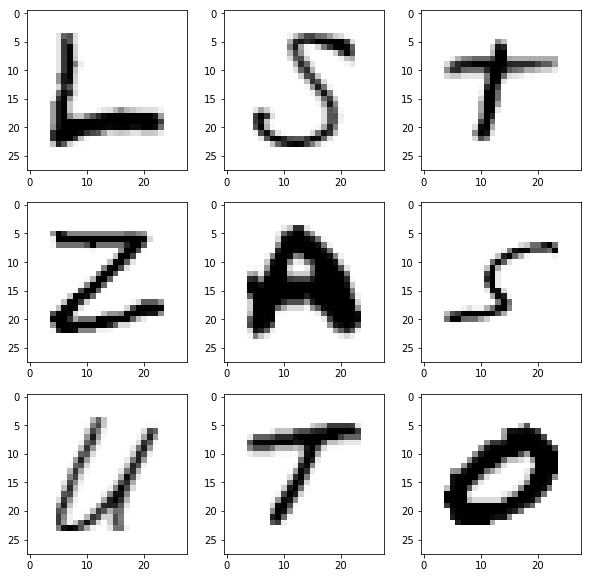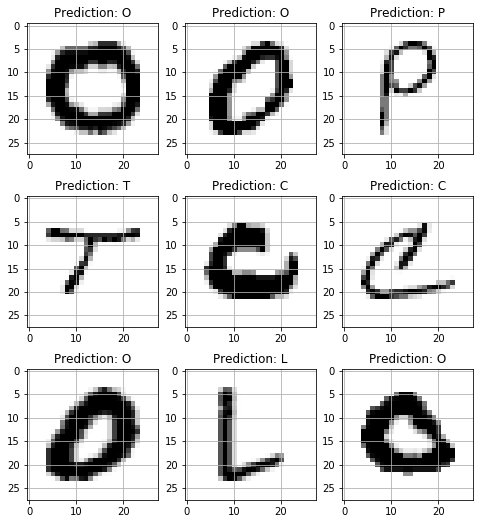• +91-9872993883
• +91-8283824812
• info@ris-ai.com

# Handwritten Character Recognition with Python

Handwritten Character Recognition with Python allows the computer to turn handwriting into a readable format. It is a field of research in Artificial Intelligence, Computer Vision and Pattern Recognition and is used to recognize text inside images, such as scanned documents and photos.
In this Machine Learning project, we will recognize handwritten characters i.e English alphabets from A-Z. We are going to achieve this by modeling a neural network that is trained over a dataset containing images of alphabets.

## Steps to implement the Handwritten Character Recognition:

1. Import the libraries and load the dataset.
2. Preprocess data
3. Create and Train the model
4. Evaluate the model
5. Create GUI to predict characters

#### First we import all necessary libraries :

In :
from keras.datasets import mnist
import matplotlib.pyplot as plt
import cv2
import numpy as np
from keras.models import Sequential
from keras.layers import Dense, Flatten, Conv2D, MaxPool2D, Dropout
from keras.callbacks import ReduceLROnPlateau, EarlyStopping
from keras.utils import to_categorical
import pandas as pd
import numpy as np
from sklearn.model_selection import train_test_split
from keras.utils import np_utils
import matplotlib.pyplot as plt
from tqdm import tqdm_notebook
from sklearn.utils import shuffle

Using TensorFlow backend.
/home/webtunix/.local/lib/python3.5/site-packages/tensorflow/python/framework/dtypes.py:493: FutureWarning: Passing (type, 1) or '1type' as a synonym of type is deprecated; in a future version of numpy, it will be understood as (type, (1,)) / '(1,)type'.
_np_qint8 = np.dtype([("qint8", np.int8, 1)])
/home/webtunix/.local/lib/python3.5/site-packages/tensorflow/python/framework/dtypes.py:494: FutureWarning: Passing (type, 1) or '1type' as a synonym of type is deprecated; in a future version of numpy, it will be understood as (type, (1,)) / '(1,)type'.
_np_quint8 = np.dtype([("quint8", np.uint8, 1)])
/home/webtunix/.local/lib/python3.5/site-packages/tensorflow/python/framework/dtypes.py:495: FutureWarning: Passing (type, 1) or '1type' as a synonym of type is deprecated; in a future version of numpy, it will be understood as (type, (1,)) / '(1,)type'.
_np_qint16 = np.dtype([("qint16", np.int16, 1)])
/home/webtunix/.local/lib/python3.5/site-packages/tensorflow/python/framework/dtypes.py:496: FutureWarning: Passing (type, 1) or '1type' as a synonym of type is deprecated; in a future version of numpy, it will be understood as (type, (1,)) / '(1,)type'.
_np_quint16 = np.dtype([("quint16", np.uint16, 1)])
/home/webtunix/.local/lib/python3.5/site-packages/tensorflow/python/framework/dtypes.py:497: FutureWarning: Passing (type, 1) or '1type' as a synonym of type is deprecated; in a future version of numpy, it will be understood as (type, (1,)) / '(1,)type'.
_np_qint32 = np.dtype([("qint32", np.int32, 1)])
/home/webtunix/.local/lib/python3.5/site-packages/tensorflow/python/framework/dtypes.py:502: FutureWarning: Passing (type, 1) or '1type' as a synonym of type is deprecated; in a future version of numpy, it will be understood as (type, (1,)) / '(1,)type'.
np_resource = np.dtype([("resource", np.ubyte, 1)])


In :
data = pd.read_csv('A_Z Handwritten Data.csv').astype('float32')


## Handwritten Character Recognition with Python

#### Split data X-Our data and y-Predicted label

In :
X = data.drop('0',axis = 1)
y = data['0']


#### Reshaping the data in csv file so that it can be displayed as an image...

In :
train_x, test_x, train_y, test_y = train_test_split(X, y, test_size = 0.2)
train_x = np.reshape(train_x.values, (train_x.shape, 28,28))
test_x = np.reshape(test_x.values, (test_x.shape, 28,28))

print("Train data shape: ", train_x.shape)
print("Test data shape: ", test_x.shape)

Train data shape:  (297960, 28, 28)
Test data shape:  (74490, 28, 28)


#### Dictionary for getting characters from index values...

In :
word_dict = {0:'A',1:'B',2:'C',3:'D',4:'E',5:'F',6:'G',7:'H',8:'I',9:'J',10:'K',11:'L',12:'M',13:'N',14:'O',15:'P',16:'Q',17:'R',18:'S',19:'T',20:'U',21:'V',22:'W',23:'X', 24:'Y',25:'Z'}


#### Plotting the number of alphabets in the dataset...

In :
train_yint = np.int0(y)
count = np.zeros(26, dtype='int')
for i in train_yint:
count[i] +=1

alphabets = []
for i in word_dict.values():
alphabets.append(i)

fig, ax = plt.subplots(1,1, figsize=(10,10))
ax.barh(alphabets, count)

plt.xlabel("Number of elements ")
plt.ylabel("Alphabets")
plt.grid()
plt.show()#### Shuffling the data ...

In :
shuff = shuffle(train_x[:100])
fig, ax = plt.subplots(3,3, figsize = (10,10))
axes = ax.flatten()

for i in range(9):
axes[i].imshow(np.reshape(shuff[i], (28,28)), cmap="Greys")
plt.show()#### Reshaping the training & test dataset so that it can be put in the model...

In :
train_X = train_x.reshape(train_x.shape,train_x.shape,train_x.shape,1)
print("New shape of train data: ", train_X.shape)

test_X = test_x.reshape(test_x.shape, test_x.shape, test_x.shape,1)
print("New shape of train data: ", test_X.shape)

New shape of train data:  (297960, 28, 28, 1)
New shape of train data:  (74490, 28, 28, 1)


#### Converting the labels to categorical values...

In :
train_yOHE = to_categorical(train_y, num_classes = 26, dtype='int')
print("New shape of train labels: ", train_yOHE.shape)

test_yOHE = to_categorical(test_y, num_classes = 26, dtype='int')
print("New shape of test labels: ", test_yOHE.shape)

New shape of train labels:  (297960, 26)
New shape of test labels:  (74490, 26)


#### CNN model..

In :
model = Sequential()

reduce_lr = ReduceLROnPlateau(monitor='val_loss', factor=0.2, patience=1, min_lr=0.0001)
early_stop = EarlyStopping(monitor='val_loss', min_delta=0, patience=2, verbose=0, mode='auto')

history = model.fit(train_X, train_yOHE, epochs=1, callbacks=[reduce_lr, early_stop],  validation_data = (test_X,test_yOHE))

model.summary()
model.save(r'model_hand.h5')

Train on 297960 samples, validate on 74490 samples
Epoch 1/1
297960/297960 [==============================] - 430s 1ms/step - loss: 1.1870 - acc: 0.8983 - val_loss: 0.0836 - val_acc: 0.9761
_________________________________________________________________
Layer (type)                 Output Shape              Param #
=================================================================
conv2d_4 (Conv2D)            (None, 26, 26, 32)        320
_________________________________________________________________
max_pooling2d_4 (MaxPooling2 (None, 13, 13, 32)        0
_________________________________________________________________
conv2d_5 (Conv2D)            (None, 13, 13, 64)        18496
_________________________________________________________________
max_pooling2d_5 (MaxPooling2 (None, 6, 6, 64)          0
_________________________________________________________________
conv2d_6 (Conv2D)            (None, 4, 4, 128)         73856
_________________________________________________________________
max_pooling2d_6 (MaxPooling2 (None, 2, 2, 128)         0
_________________________________________________________________
flatten_2 (Flatten)          (None, 512)               0
_________________________________________________________________
dense_4 (Dense)              (None, 64)                32832
_________________________________________________________________
dense_5 (Dense)              (None, 128)               8320
_________________________________________________________________
dense_6 (Dense)              (None, 26)                3354
=================================================================
Total params: 137,178
Trainable params: 137,178
Non-trainable params: 0
_________________________________________________________________


#### Displaying the accuracies & losses for train & validation set...

In :
print("The validation accuracy is :", history.history['val_acc'])
print("The training accuracy is :", history.history['acc'])
print("The validation loss is :", history.history['val_loss'])
print("The training loss is :", history.history['loss'])

The validation accuracy is : [0.9760907504363002]
The training accuracy is : [0.8983185662505034]
The validation loss is : [0.08358431425431195]
The training loss is : [1.1870133685692619]


#### Making model predictions...

In :
pred = model.predict(test_X[:9])
print(test_X.shape)

(74490, 28, 28, 1)


#### Displaying some of the test images & their predicted labels...

In :
fig, axes = plt.subplots(3,3, figsize=(8,9))
axes = axes.flatten()

for i,ax in enumerate(axes):
img = np.reshape(test_X[i], (28,28))
ax.imshow(img, cmap="Greys")
pred = word_dict[np.argmax(test_yOHE[i])]
ax.set_title("Prediction: "+pred)
ax.grid()